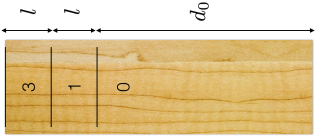# Shuffleboard physics (I)

Table shuffleboard is a game (usually found in higher quality bars) in which a heavy puck is glided down a long table toward an end zone. Points are scored by getting the puck to come to rest as close to the edge as possible, the closer the puck, the higher the reward.

If the puck slides off the end of the table, however, the player gets zero points. In the table shown below, three points are awarded for the far zone, one for the near zone, and zero if the puck doesn't cross the first line.Crudely, the difficulty $D$ of making it into each zone is proportional to $1/\Delta s$, the range of allowable speeds between which the puck still stop inside the given zone. For example, if the puck is released with speed $s_3^\textrm{max}$, it will stop just before falling off the end of the table, and if released with speed $s_3^\textrm{min}$, it will stop just inside the three point zone, and $\Delta s_3 = s_3^\textrm{max} - s_3^\textrm{min}$.

Suppose the puck and the table have a coefficient of kinetic friction given by $\mu_k$, and that the puck is pulled down by the acceleration due to gravity, $g$. How much more difficult is it to get the puck to stop in the three point zone than in the one point zone? In other words, find

$\frac{D_3}{D_1} = \frac{\Delta s_1}{\Delta s_3}$

Details

• $d_0=15$ m
• $l=5$ m
×